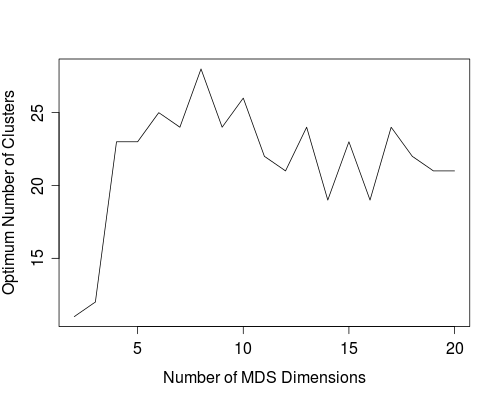# Category Archives: MDS

## Harappa Genome Similarity MDS/Dendrogram

I computed the IBS similarity matrix for the Harappa participants HRP0001 to HRP0080 over 500,000 SNPs. This is exactly the same thing as the genome-wide gene comparison at 23andme.

Then, I converted the similarity matrix to a dissimilarity/distance matrix with the standard formula:

dij = sqrt(2 - 2 * sij)

where sij is the similarity between individuals i and j and dij is the distance/dissimilarity between the two.

Using the dissimilarity matrix, I classified all the participants (excluding close relatives) using hierarchical clustering with complete linkage. You can see the dendrogram below.Then I used the same dissimilarity matrix to calculate 6-dimensional MDS. You can see the MDS plots below. The numbers on the plots are your Harappa IDs.

As you can see I (HRP0001) and my sister (HRP0035) are far away in the first four dimensions.

I'll let you guys speculate on what each dimension represents.

Now why create an MDS this way instead of directly using Plink's MDS functionality? Well, I needed to check if I could do it using only the similarity matrix because that would be really useful for something else. Tune in on my other blog for more later this week.

## Ref 2 South Asians + Harappa MDS Clusters

Why do MDS clusters when we already did PCA-based clustering for this data?

You guys probably know about Dienekes' Clusters Galore approach. The way it works is that varying the number of MDS dimensions used you compute the number of clusters inferred (done using Mclust) and use the number of MDS dimensions which give you the maximum number of clusters.

This sounded a little unsatisfactory for me. So I ran an experiment. I computed 100 MDS dimensions for the samples in this dataset which includes South Asians from Reference II as well as 38 Harappa participants. Then I kept 2,3,4,...,100 dimensions and ran NNClean (to get initial noise/outlier estimate) and Mclust on them.

This first graph shows the number of outliers NNclean computed from 586 samples.Things go crazy with NNclean when 64 or more MDS dimensions are retained since it considers most of the samples to be noise then.

Now let's look at the number of outliers identified after Mclust's clustering procedure.This shows us that probably somewhere between 8 and 65 MDS dimensions might be useful to keep.

Finally, a plot of the number of clusters inferred by Mclust versus the number of MDS dimensions used.There are two big jumps here to consider. One is around 12 MDS dimensions and the other after 52. So we are looking at an optimum number of MDS dimensions between 12 and 52. However, in that range, the number of clusters computed is fairly noisy between 18 and 26. The only pattern I can discern with some smoothed fitting is that we should likely be looking at somewhere between 20 and 30 MDS dimensions.But why choose the maximum number of clusters (26 clusters when 24 MDS dimensions are kept)? That could be the result of noise too.

Is there some other way to figure out what are the significant number of MDS dimensions to keep for population structure? It turns out there is. Patterson, Price and Reich proposed Tracy-Widom statistics for Principal Component Analysis in their paper "Population Structure and Eigenanalysis". We also know that the MDS analysis we are performing is the classical metric MDS which is in some ways equivalent to a PCA. Looking at the Tracy Widom stats then, we see that about 25 eigenvalues are significant. Thus, keeping 24 MDS dimensions to maximum the number of clusters seems defensible.

Finally, here are the clustering results.

## Ref1 South Asian + Harappa MDS MClust

Now I am going nuts on this dataset consisting of South Asians (minus Kalash and Hazara) from Reference I and some Harappa participants, but I promise this is the last item on this specific data. I will however do similar analyses some time after integrating all the new South Asian samples I have gotten (via project participation as well as from research data).

I ran MDS on the data in Plink and then retaining various number of MDS dimensions, ran MClust on it. This is what Dienekes calls Clusters Galore.

The graph of number of MDS dimensions retained versus optimum number of clusters computed by Mclust is as follows:The maximum number of clusters (28) are inferred with 8 MDS dimensions. So I posted the clustering results for 8 MDS dimensions + 28 clusters.

Some observations on the clusters:

1. 56 of the 62 Gujaratis are in cluster CL1 and the remaining 6 are in CL5. Both are Gujarati-only clusters. Let's see where the Harappa Gujaratis fall next time I do this analysis,
2. CL2 has an Andhra Reddy, Caribbean Indians, a Keralan, a few Gujaratis-B, and a third of the Singapore Indians.
3. Gujaratis-B are a varied lot spread out into CL3, CL7, CL2, CL8, CL4, CL6, and CL15, but half are in CL3.
4. CL6 has a lot of the South Indian Brahmins
5. Burusho are isolated
6. Punjabis from the project seem to be divided among CL7, CL8 and CL15.

I also posted the results for 20 MDS dimensions resulting in 21 clusters.